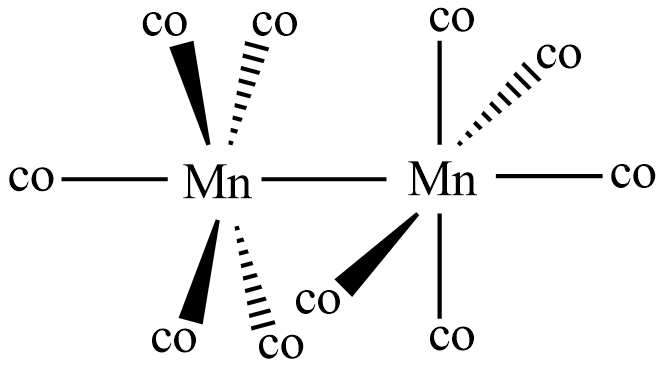# ${\text{M}}{{\text{n}}_{\text{2}}}{\left( {{\text{CO}}} \right)_{{\text{10}}}}$ is an organometallic compound due to the presence of:(A)Mn-Mn bond(B)Mn-C bond(C)Mn-O bond(D)C-O bondVerified
175.5k+ views
Hint: Organometallic compounds are formed by bonding of metals with organic moiety. Some of the d-block metals form coordination compounds with organic molecules known as organometallic compounds.

Complete Step-by-step solution:
Organometallic compounds consist of some features to be defined or regarded as organometallic compounds. The main properties include 1) There should be at least one metal to carbon atom bond in the compound, where the carbon is from organic moiety. 2) The structure of the compound has metal to metal bond.So in the given compound ${\text{M}}{{\text{n}}_{\text{2}}}{\left( {{\text{CO}}} \right)_{{\text{10}}}}$ called as manganese carbonyl or dimanganese decacarbonyl, there occurs bonds between manganese and the carbon atoms of carbonyl group derived from sodium benzophenone ketyl. If we observe the structure of the manganese carbonyl it is seen having a bond between two manganese atoms. And also each metal atom is bonded to five carbonyl groups. This proves that ${\text{M}}{{\text{n}}_{\text{2}}}{\left( {{\text{CO}}} \right)_{{\text{10}}}}$ is an organometallic compound as it has metal-carbon atom bond.

So the answer for the above question is option (B) Mn-C bond.

Additional information: But some compounds even though having metal-carbon bonds are not considered as organometallic compounds because the carbon atom is not from an organic entity. The best examples for such compounds are Iron carbide ${\text{F}}{{\text{e}}_{\text{3}}}{\text{C}}$ and potassium ferrocyanide ${\text{KF}}{{\text{e}}_{\text{2}}}{\left( {{\text{CN}}} \right)_{\text{6}}}$. The best example for well-known organometals widely used as reagents in several organic reactions is Grignard reagent.

Note: In manganese carbonyl the carbonyl groups are called ligands and there are no bridging ligands in it. The two ${\text{MnC}}{{\text{O}}_{\text{5}}}$ parts occur in a staggered position in its structure to be stable.